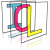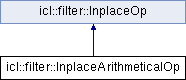Image Component Library (ICL)
icl::filter::InplaceArithmeticalOp Class Reference

Inplace implementation for arithmetical operations. More...

`#include <InplaceArithmeticalOp.h>`

Inheritance diagram for icl::filter::InplaceArithmeticalOp:## Public Types

enum  optype {
addOp =0, subOp =1, mulOp =2, divOp =3,
sqrOp =10, sqrtOp =11, lnOp =12, expOp =13,
absOp =14
}
Optypes specify the certain operation. More...

## Public Member Functions

InplaceArithmeticalOp (optype t, icl64f value=0)
Create new instance with given operator type and optional value. More...

virtual core::ImgBaseapply (core::ImgBase *src)
applys the operation inplace on an input image More...

icl64f getValue () const
returns the current value More...

void setValue (icl64f val)
sets the current value More...

optype getOpType () const
returns the current operator type More...

void setOpType (optype t)
sets the current operator type More...Public Member Functions inherited from icl::filter::InplaceOp
InplaceOp ()
Create a new Inplace op (ROI-only flag is set to true) More...

void setROIOnly (bool roiOnly)
setup the operation to work on the input images ROI only or not More...

bool getROIOnly () const
returns whether operator is in "roi-only" mode or not More...

## Private Attributes

optype m_eOpType
operator type More...

icl64f m_dValue
value More...

## Detailed Description

Inplace implementation for arithmetical operations.

Current supported operations: "+","-","*","/","^2","sqrt2, "ln", "exp" and "abs".
Currently no IPP-Optimization is available

## ◆ optype

Optypes specify the certain operation.

Enumerator

add a constant value to each pixel

subOp

substract a constant value from each pixel

mulOp

multiply each pixel by a constant value

divOp

divide each pixle by a constant value

sqrOp

squares each pixel

sqrtOp

calculates the square root of each pixel

lnOp

calculates the natural logarithm of each pixel

expOp

calculates the exponential function for each pixel

absOp

calculates the absolute value for each pixel

## ◆ InplaceArithmeticalOp()

 icl::filter::InplaceArithmeticalOp::InplaceArithmeticalOp ( optype t, icl64f value = `0` )
inline

Create new instance with given operator type and optional value.

Parameters
 t operator type value 2nd operand for the operations. Some operation like "ln" or "abs" do not need this operand, so it can be omitted.

## ◆ apply()

 virtual core::ImgBase* icl::filter::InplaceArithmeticalOp::apply ( core::ImgBase * src )
virtual

applys the operation inplace on an input image

Implements icl::filter::InplaceOp.

## ◆ getOpType()

 optype icl::filter::InplaceArithmeticalOp::getOpType ( ) const
inline

returns the current operator type

## ◆ getValue()

 icl64f icl::filter::InplaceArithmeticalOp::getValue ( ) const
inline

returns the current value

## ◆ setOpType()

 void icl::filter::InplaceArithmeticalOp::setOpType ( optype t )
inline

sets the current operator type

## ◆ setValue()

 void icl::filter::InplaceArithmeticalOp::setValue ( icl64f val )
inline

sets the current value

## ◆ m_dValue

 icl64f icl::filter::InplaceArithmeticalOp::m_dValue
private

value

## ◆ m_eOpType

 optype icl::filter::InplaceArithmeticalOp::m_eOpType
private

operator type

The documentation for this class was generated from the following file: